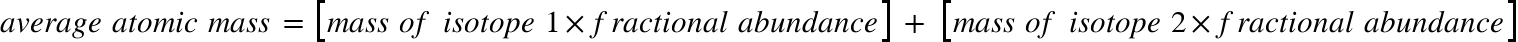Chemistry Isotopes Solution: Antimony has many uses, for example, in infrared d...

🤓 Based on our data, we think this question is relevant for Professor Roland's class at UCSC.

# Solution: Antimony has many uses, for example, in infrared devices and as part of an alloy in lead storage batteries. The element has two naturally occurring isotopes, one with mass 120.904 amu and the other with mass 122.904 amu. (b) Use the atomic mass of antimony from the periodic table to calculate the natural abundance of each isotope.

Problem

Antimony has many uses, for example, in infrared devices and as part of an alloy in lead storage batteries. The element has two naturally occurring isotopes, one with mass 120.904 amu and the other with mass 122.904 amu.

(b) Use the atomic mass of antimony from the periodic table to calculate the natural abundance of each isotope.

Solution

Average atomic mass of an element is given by:We have the following masses of two antimony isotopes:

Isotope 1 = 120.904 amu, Let’s say its frac. Abund. Is x

Isotope 2 = 122.904 amu, it’s frac. Abundance is then (1-x)

We can find the average atomic mass of antimony from the periodic table. It is 121.760 amu.

We can put all these values into our formula:View Complete Written Solution